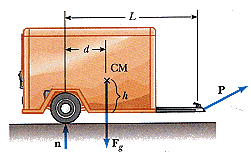# Forces, Acceleration Hard Stuff (HELP!)

[SOLVED] Forces, Acceleration....Hard Stuff (HELP!)A trailer with loaded weight Fg is being pulled by a vehicle with a force P. The trailer is loaded such that its center of mass is located as shown. Neglect the force of rolling friction and let a represent the x component of the acceleration of the trailer.

(a) Find the vertical component of P in terms of the given parameters. (Use F_g for Fg, a, d, L, h, and g as appropriate in your equation.)

I have no idea on how to use come up with an expression that involves acceleration. In matter of fact I do not know where to start because this looks confusing. Can anyone give me advice?

Thanks,
Kevin

#### Attachments

Hootenanny
Staff Emeritus
Gold Member
What can you deduce about the total torque about any point on the trailer?

Here is how I found the correct answer:

First, break down force P into two components: Px and Py

Second, consider the center of mass to be your axis point.

Third, derive an equation for the the net forces in y-components, $$\sum$$Fy, and let that equal to 0.

Py + n - Fg = 0 (1)

Fourth, derive an equation for the only force in the x-component.

Px = m*a

(and since the force of gravity on the whole truck is mg, than m = Fg/g)
So...

Px = (Fg/g)*a (2)

Fifth, derive an equation for the net torques, $$\sum$$$$\tau$$ acting on the center of mass, which when summed, should equal 0.

Py*(L-d) + Px*h -n*d = 0 (3)

(Both Px and Py produce torque in the same direction so therefore they should be added and then subtracted by the normal force, which is producing torque in the opposite direction.)

Now using equation (3), rearrange the equation so that you have it in terms of n.

n = [(Px*h) + Py*(L-d)]*(1/d)

Since you know Px from equation (2), then you can plug that into the equation above.

n = [(Fg*a*h/g) + Py*(L-d)]*(1/d)

Simplified a bit further...

n = [(Fg*a*h) + Py*g*(L-d)]/(d*g)

Now using the n above, rearrange equation (1) in terms of Py, and then plug in your derived expression for n, and you have the following:

Py = Fg - [(Fg*a*h) + Py*g*(L-d)]/(d*g)

Simplify a bit so you have your Py on one side....

Py*d*g + Py*g*(L-d) = Fg*d*g - Fg*a*h

When you pull out Pyg and divide L on both sides....

Py*g = Fg*(d*g - a*h)/L

Py = (Fg/L)*(d - a*h/g)

I hope this helps others who were stuck on this problem.

Kevin